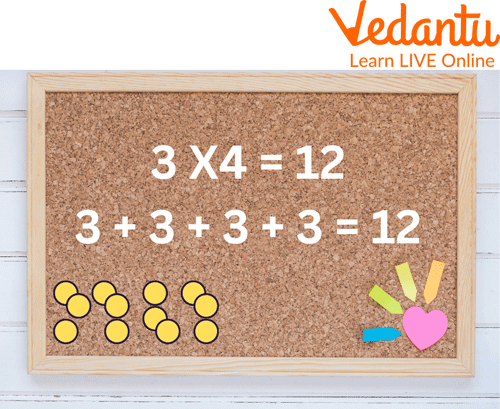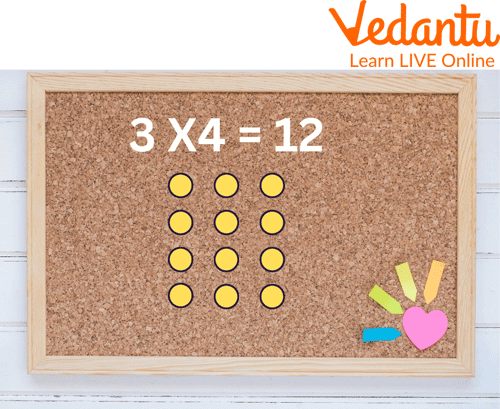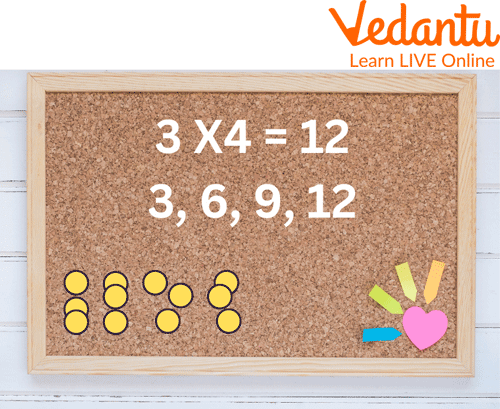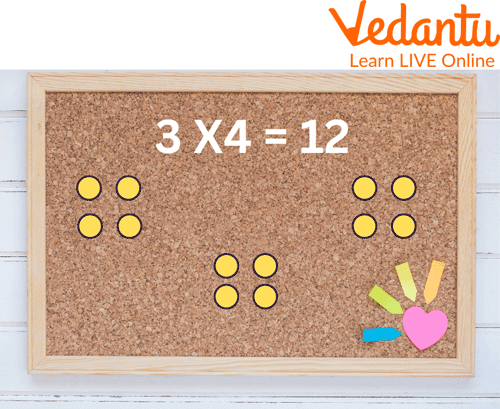Courses
Courses for Kids
Free study material
Free LIVE classes
More

# Multiplication Strategies## Introduction to Multiplication Strategies

Hello kids. Do you struggle with multiplication? Let's make multiplication easier for you. We all know how to multiply, divide, add or subtract, but we don’t know the easier way to make the calculation fast and accurate. So, today we will learn about some maths multiplication strategies. Different multiplication strategy charts will make teaching multiplication easy and fun for you. Using these multiplication strategies, you can solve any problem quickly and accurately. Also, these strategies are conceptual and work with any multiplication problem. Therefore, it deepens your understanding of multiplication, which leads to tremendous success with word problems and division.

## Different Multiplication Strategies

This strategy is the simplest of all. This strategy is the foundational one, which will further help to understand the other strategies. It helps with conceptual understanding, even though it feels tedious and time-Consuming.

For example,

If you have to find products of 5 and 3, you can do it in two ways: add 5 three times or add 3 five times.• ## Arrays

This strategy helps “decompose” a more difficult multiplication problem into smaller ones. This multiplication strategy makes it easy to visualise the decompositions. In this, we have to make rows and columns for multiplication.

For Example: Find a product of $3 \times 4$

Solution: We can be decomposed $3 \times 4$ into $2 \times 3+2 \times 3$.

$3 \times 4=2 \times 3+2 \times 3$ (make 2 rows and 3 columns for each and then add)

$=6+6$

$=12$

Another method is to form an array compromising 4 rows and 3 columns.Array Method

• ## Using 1s, 2s, and 5s

The decomposing array strategy immediately followed the following strategy. With this approach, we solve for unknown facts by using the known ones, usually 1s, 2s, and 5.

For Example: Find the product of 8 X 4.

For Example: Find the product of $8 \times 4$.

Ans: 8 groups of $3=5+2+1$ group of 4.

$5 \times 4 =20$

$2 \times 4 =8$

$1 \times 4 =4$

$20+8+4 =32 .$

Therefore, the answer of $8 \times 4$ is 32.

• ## Skip Counting With a Twist

Skip counting can be used for creating equal groups and arrays, just as repeated addition. For the example 4X3, this can be solved by skipping count by 4 three times or by 3 four times.

For Example: Find the product of 4 X 3

Solution: By skipping the count by 4 three times we will get 4, 8, 12.

Or by skipping the count by 3 four times we will get 3, 6, 9, 12.Skip Counting

• ## Add a Group or Take Away a Group

This Multiplication strategy starts with making groups. This is one of the simplest strategies to start with as a kid. We start with making groups and then, according to the requirement we add a group or take away a group.

For Example: Find the Product of $3 \times 4$

Ans: Make a group of 4 round boxes each. Let us make 3 groups named A, B, and C with 4 round boxes each.Making a group strategy

Now, let us solve this by adding a group strategy. We know that $2 \times 4=8$ Or 2 groups of $4=8$. Add another group of 4 to solve 3 groups of 4 .

2 groups of $4+$ one more group of 4

$8+4=12 \text {. }$

The answer to $3 \times 4$ is 12 .

This can be done by taking away a group as well.

## Solved Examples on Easy Methods for Multiplication

Q1. Find the product of 2 x 3.

Sol. By using repeated addition, we will solve this question. We will get the answer by adding 2 three times or adding 3 two times.

2+2+2 or 3+3

6 or 6

So the answer is 2 X 3 = 6.

Q2. Find the product of 5 X 3.

Sol: By skipping the count by 5 three times, we will get 5, 10, 15.

Or by skipping the count by 3 five times, we will get 3, 6, 9, 12, 15.

## Practice Questions

Some practice questions from the multiplication strategies pdf are below:

Q1. What is $3 \times 6$?

Ans. 18

Q2. What are 6 times 5?

Ans 30

Q3. What will be the product if 5 is multiplied by 3?

Ans. 15

## Summary

Multiplication in mathematics is a technique for determining the sum of two or more numbers. It is a fundamental mathematical operation that we apply daily. Multiplying means combining like groups. The quantity of items in the group expands as we multiply. Parts of a multiplication problem include the two factors and the product. Here we have learnt various maths multiplication strategies and easy multiplication methods, making teaching multiplication simple and fun for kids. These strategies will help solve other mathematical problems. We can also go through the multiplication strategies pdf for concept clarity.

Last updated date: 24th Sep 2023
Total views: 81.9k
Views today: 0.81k

## FAQs on Multiplication Strategies

1. How is multiplication useful in our daily life?

Throughout adulthood, multiplication is a daily necessity for tasks like figuring out price breaks, doubling recipes, and sharing expenses. Children who have already mastered their tables find it simpler to solve math problems and perform mental calculations.

2. What is the difference between multiplication and division?

In multiplication, we combine groups of equal sizes, while in the division, we split or separate the given number into equal groups. Multiplication is the product of two or more numbers where the numbers that are multiplied are the factors, and the result is termed the product. In division, the number that is divided is called the dividend, the number which divides the dividend is called the divisor, and the result is the quotient.

3. How is multiplication related to addition?

Multiplication represents the basic idea of repeated addition of the same number. It simplifies the task of repeated addition. For example, if there are 3 packs of pencils and each pack has 6 pencils, let us find the total number of pencils. We can solve this question by adding 6 + 6 + 6 = 18 pencils. If we use multiplication to solve this problem, we need to multiply the number of packs by the number of pencils in each pack. This means $3 \times 6 = 18$ pencils.